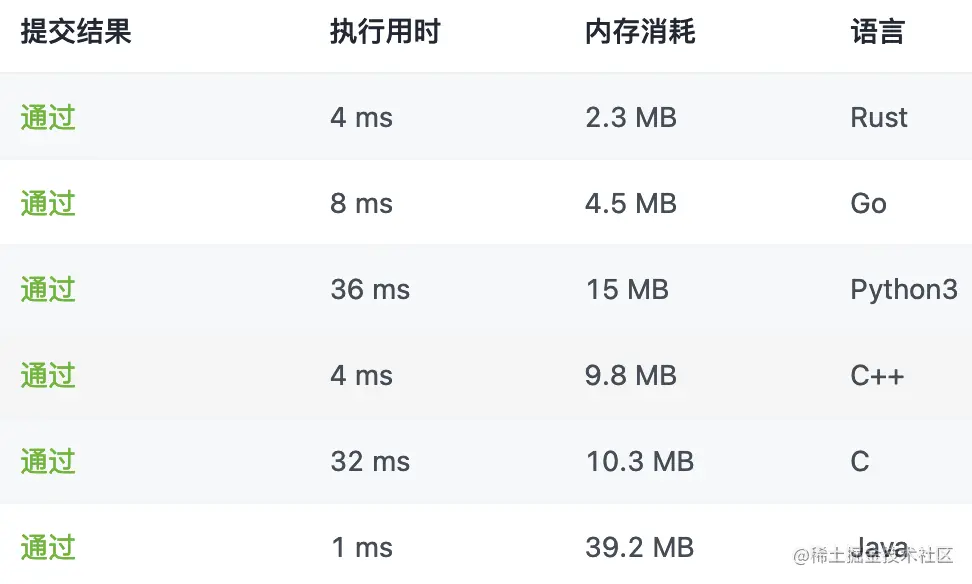# 1313. Unzip the coding list (Java / C / C + + / Python / go / trust)

2022-02-01 15:49:19

This is my participation 11 The fourth of the yuegengwen challenge 11 God , Check out the activity details ：2021 One last more challenge

welcome 【 give the thumbs-up 】【 Collection 】【 Comment on 】~
It's not hard to give up , But persistence must be cool ~
I hope all of us can make a little progress every day ~
This paper is written by The white hat of the second leader ：https://le-yi.blog.csdn.net/ Original blog ~

# 1313. Decompress the encoding list ：

Give you a compressed list of integers encoded by run length `nums` .

Consider each pair of two adjacent elements `[freq, val] = [nums[2*i], nums[2*i+1]]` （ among `i >= 0` ）, Each pair indicates that there are... In the sub list after decompression `freq` The values are `val` The elements of , You need to connect all sub lists from left to right to generate the unzipped list .

# Examples 1：

`````` Input ：
nums = [1,2,3,4]

Output ：
[2,4,4,4]

explain ：
The first pair  [1,2]  Represents the  2  The frequency of occurrence is  1, So generate an array  .
Second pairs  [3,4]  Represents the  4  The frequency of occurrence is  3, So generate an array  [4,4,4].
Finally, connect them together   + [4,4,4] = [2,4,4,4].
Copy code ``````

# Examples 2：

`````` Input ：
nums = [1,1,2,3]

Output ：
[1,3,3]
Copy code ``````

# Tips ：

• 2 <= nums.length <= 100
• nums.length % 2 == 0
• 1 <= nums[i] <= 100

# analysis

• This algorithm problem really doesn't have any special skills , Just simulate according to the meaning of the topic .

• For most languages, there is support for dynamic data structures , But there is one thing to note about dynamic data structures , When dynamically adding elements , Dynamic expansion occurs , Content copying may also occur .

• Some of the solutions of the second leader's problems are traversed first , Calculate the total length , This overhead can offset the overhead of dynamic expansion using dynamic data structures .

## java

``````class Solution {
public int[] decompressRLElist(int[] nums) {
int len = 0;
for (int i = 0; i < nums.length; i += 2) {
len += nums[i];
}
int[] ans = new int[len];
for (int i = 0, j = 0; i < nums.length; i += 2) {
for (int k = 0; k < nums[i]; ++k) {
ans[j++] = nums[i + 1];
}
}
return ans;
}
}
Copy code ``````

## c

``````/** * Note: The returned array must be malloced, assume caller calls free(). */
int* decompressRLElist(int* nums, int numsSize, int* returnSize){
*returnSize = 0;
for (int i = 0; i < numsSize; i += 2) {
*returnSize += nums[i];
}
int *ans = malloc(*returnSize * sizeof(int));
for (int i = 0, *p = ans; i < numsSize; i += 2) {
while (nums[i]--) {
(*p++) = nums[i + 1];
}
}
return ans;
}
Copy code ``````

## c++

``````class Solution {
public:
vector<int> decompressRLElist(vector<int>& nums) {
vector<int> ans;
for (int i = 0, l = nums.size(); i < l; i += 2) {
ans.insert(ans.end(), nums[i], nums[i + 1]);
}
return ans;
}
};
Copy code ``````

## python

``````class Solution:
def decompressRLElist(self, nums: List[int]) -> List[int]:
return [nums[i + 1] for i in range(0, len(nums), 2) for _ in range(nums[i])]

Copy code ``````

## go

``````func decompressRLElist(nums []int) []int {
l := 0
for i := 0; i < len(nums); i += 2 {
l += nums[i]
}
ans := make([]int, l)
for i, j := 0, 0; i < len(nums); i += 2 {
for k := 0; k < nums[i]; k, j = k+1, j+1 {
ans[j] = nums[i+1]
}
}
return ans
}
Copy code ``````

## rust

``````impl Solution {
pub fn decompress_rl_elist(nums: Vec<i32>) -> Vec<i32> {
(0..nums.len()).step_by(2).flat_map(|i|{
[nums[i + 1]].repeat(nums[i] as usize).into_iter()
}).collect()
}
}
Copy code ``````